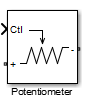Main Content

# Potentiometer

Simulink controlled potentiometer

• Library:
• RF Blockset / Circuit Envelope / Junctions

•## Description

The Potentiometer block models a variable resistor. The Potentiometer block uses Simulink® signal as input control signal to vary the resistance between the positive and the negative terminals.

The equations for the potentiometer voltage–current relationships when the control signal varies between zero and one are:

• Linear, Logarithmic, Antilog — Electrical characteristic of the potentiometer.

• `Ctl` — Simulink signal

• `Ron` — Minimum resistance of the potentiometer

• `Roff` — Maximum resistance of the potentiometer

## Parameters

expand all

Minimum input resistance of the potentiometer, specified as a positive scalar in ohms.

Data Types: `double`

Maximum input resistance of potentiometer, specified as a positive scalar in ohms. This value must be greater than the minimum resistance.

Data Types: `double`

Curve type of the potentiometer, specified as `Linear`, `Logarithmic`, `Antilog`.

Data Types: `char`

Percentage of resistance at half wiper for logarithmic and antilog potentiometer types, specified as a positive scalar. The valid values depend on the potentiometer type:

• `Logarithmic` - Greater than 0 and less than 50. The default is 20.

• `Antilog` - Greater than 50 and less than 100. The default is 80.

#### Dependencies

To enable this parameter, select `Logarithmic` or `Antilog` in Potentiometer type.

Data Types: `double`

## See Also

Introduced in R2015b

## Support

#### Model RF Power Amplifiers and Increase Transmitter Linearity with DPD Using MATLAB

Download white paper